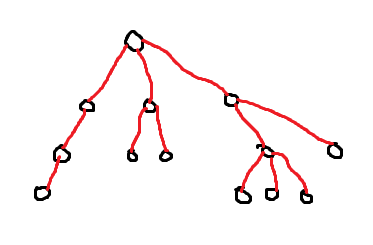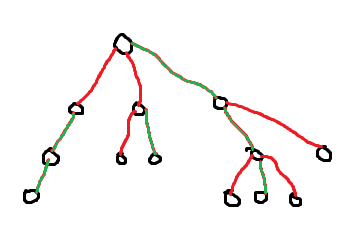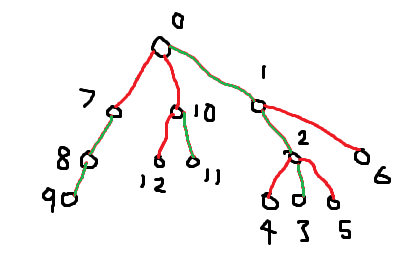# (Tutorial) Heavy-Light Decomposition

As requested by @saurabhshadow, @anon53253486 (kind of), and probably others

Hi! If you’re frantically looking for an implementation for some contest, here’s my implementation being used to solve this problem. The struct hld is the important part. You can find a quick note on how to modify it for edge queries/updates below.

For the rest of you, let’s do this!

This is a specific algorithm I’m going to talk about. So before I begin the explanation, let me describe what we’re actually trying to do here.

# The motivation (path queries)

Heavy-light decomposition is kind of a scary name, but it’s really cool for what it does. Take some classic segment tree problem with two types of queries:

• Compute the XOR of values on the subarray from l to r
• Update the value at position i to x

Now, anyone familiar with a segment tree will recognize that it can be easily applied to solve this. But what if we moved the problem from queries on an array to queries on a tree? Let’s reformulate the queries to be:

• Compute the XOR of values on the path from u to v
• Update the value at vertex i to x

which is exactly this problem from USACO, and the problem I’ll be using as an example for the rest of this tutorial. To note, its editorial also provides a nice description of HLD.

# Prerequisites

This is a relatively advanced topic, and this tutorial is not meant for beginners. Before reading this blog, you should know:

• Basic tree algorithms (such as dfs)
• Segment trees (at the very least, what their purpose is), with lazy propagation
• Binary lifting (useful for my implementation, other implementations may not use it)
• Lowest common ancestor (LCA)

# The concept

So… what? How do we turn a tree into something we can use a segment tree on? Well, we can’t exactly. What we can do is turn a tree into a bunch of paths, each of which we can build a segment tree on. That’s an incredibly vague description, so let me describe what I mean.

Consider this poorly drawn tree (rooted arbitrarily):What we’re going to do is, for each vertex that isn’t a leaf, find the edge that leads to the child with the largest subtree (breaking ties arbitrarily). I’ll color these edges green:(blame MS Paint for the colors looking weird). We’ll call these special edges “heavy” edges (because they lead to the “heaviest” child) and the rest of them “light” edges. This turns out to be super nice to work with because the heavy edges always form “vertical chains” - paths that go from some vertex to an ancestor of it. Brushing aside the implementation for a second, let’s assume we’ve built a segment tree on each of these vertical chains. Now the key idea about this setup is that any path on the tree will pass through at most O(\log{n}) light edges. By extension, each path also passes through at most O(\log{n}) vertical chains.

We should definitely prove that. For notation, from now on I will call paths of heavy edges “heavy chains”. One useful idea for the proof of this claim is that you can break any path u -> v on a tree into two (possibly non-existent) components: the path from u up to lca(u, v) and the path from v up to lca(u, v), where lca(u, v) is the lowest common ancestor of u and v. Because lca(u, v) is an ancestor of both u and v, both of these separate paths will also be vertical chains themselves. So now let’s prove that both of these vertical chains only pass through O(\log{n}) light edges.

Proof

Consider some vertex v in some vertical chain. Let the size of its subtree be x and its parent be p. If the edge from v to p is light, then there must be some other child u of p with subtree size y, where y \geq x. Then when we move up to p, the size of p's subtree is at least x + y \geq 2x. So whenever we move up a light edge, the size of our current subtree is at least doubled. Because the size of a subtree can’t be more than n, we end up moving up a light edge at most O(\log{n}) times.

Cool. Now, since each path goes through at most O(\log{n}) light edges and heavy chains, we just need to quickly evaluate all of these. Light edges are single edges, so we can get their vertices’ values quickly. As I said before, if we build a segment tree on each heavy chain, then we can evaluate the value of the intersection between our path and each heavy chain in O(\log{n}). Thus, the total complexity is O(\log^2{n}) per query.

# My implementation

This is the fun part. I’ll break this up into sections. The tree is always rooted arbitrarily (at 0).

Finding the heavy edges

We compute subtree sizes with a simple DP: sz[v] = 1 + \sum\limits_{u \in child(v)} sz[u] where child(v) is the set of all children of v. Then we just have to take the child u with the maximum value of sz[u] of all children, for each vertex. In this dfs, I also set the depths of each vertex relative to the root, which is used later for evaluating queries.

Code

The value p denotes the parent of the current vertex v - I avoid infinite loops by avoiding re-visiting the parent. d is the current depth.

void dfs_size(int v, int p, int d) {
sz[v] = 1;
depth[v] = d;
par[v] = p;
int bigc = -1, bigv = -1;

for (int x: edges[v]) {
if (x != p) {
dfs_size(x, v, d + 1);
sz[v] += sz[x];
if (sz[x] > bigv) {
bigc = x;
bigv = sz[x];
}
}
}

bigchild[v] = bigc;
}


Finding heavy chains

Now our goal is to compute, for each vertex, the vertex at the top of the heavy chain it belongs to (which could be itself). This can be done in a DP-like fashion going down the tree. We set chain[i] to:

• chain[par[i]], if i is the heavy child of its parent
• i, otherwise.

because if i is a light child, then there’s clearly no heavy chain above it, and otherwise, it just continues the heavy chain from its parent.

Code

In this implementation, initially all chain[i] = i.

void dfs_chains(int v, int p) {
if (bigchild[v] != -1) {
chain[bigchild[v]] = chain[v];
}

for (int x: edges[v]) {
if (x != p) {
dfs_chains(x, v);
}
}
}


Building a segment tree on each heavy chain

For convenience (so I or someone else can stick a segment tree template in), I choose to use a single segment tree for everything and label the nodes so heavy chains end up together in the labeling. We do a dfs and label nodes in the order that they’re visited. The simplest way to achieve labeling like what we need is to visit big children before everything else, then the label of each big child will be exactly 1 greater than that of its parent. Here’s what this would look like on the tree above (excuse my mouse-handwriting):As you can see, for this tree (and all other trees), the labels on heavy chains form consecutive sequences of numbers, so they can be easily queried with the segment tree.

Code

Initially label_time is set to 0. The function seg_update_header is simply a wrapper for the update function in my segment tree, and that call to it sets the position at label[v] to arr[v].

void dfs_labels(long long* arr, int v, int p) {
label[v] = label_time++;

if (bigchild[v] != -1) {
dfs_labels(arr, bigchild[v], v);
}

for (int x: edges[v]) {
if (x != p && x != bigchild[v]) {
dfs_labels(arr, x, v);
}
}
}


Computing queries

The best part, of course. We will, once again, leverage the fact that the path between any u and v is the same as the path from u to lca(u, v) combined with the path from v to lca(u, v). The algorithm is essentially: go up from u (inclusive) to lca(u, v) (exclusive) by efficiently computing the values from light vertices and heavy chains, then do the same for v. The convenience of setting chain[u] = u is that we can do the same thing regardless of the edge above a vertex being light or heavy. Use the segment tree to compute the value of the path from u to chain[u] then set u := par[chain[u]] until you get to computing the vertex that’s a parent of u and directly below lca(u, v) (because we need to exclude lca(u, v)).

Wait… how do we get the vertex directly below lca(u, v) (which has to be a parent of u or v)? The smart way to do this is to use the labels we’ve already constructed because as we already know, they’re consecutive. I am not smart, so I used binary lifting to get the (dep[u] - dep[lca(u, v)] - 1)-th ancestor of u. Ultimately these have the same effect, and the binary lifting only adds another O(\log{n}) to the query because we only need it once.

Now that we have that vertex, we need to make sure we don’t accidentally include the LCA or vertices above it in the query. We can do that by checking each time, if depth[chain[v]] \leq depth[lca(u, v)]. Once this is true, we set our top node (which would usually be chain[v]) to the vertex directly below lca(u, v), which automatically means we stop next iteration since we reach lca(u, v).

Code (computing a vertical chain)

get_kth_ancestor is done with binary lifting and defined in the full implementation. Its function is to just get the k-th ancestor of the input vertex. seq_query_header is the query function for my segment tree.

/* excludes p */
long long query_chain(int v, int p) {
long long val = 0;
while (depth[p] < depth[v]) {
int top = chain[v];
if (depth[top] <= depth[p]) {
int diff = depth[v] - depth[p];
top = get_kth_ancestor(v, diff - 1);
}
val = val ^ seg_query_header(label[top], label[v]);
v = par[top];
}
return val;
}


To get the answer for the full query, we combine the answers for the two vertical chains and, additionally, include the LCA.

Code (computing a full query)

lca is done with binary lifting and defined in the full implementation.

long long query(int u, int v) {
int lc = lca(u, v);
long long val = query_chain(u, lc) ^ query_chain(v, lc);
val = val ^ seg_query_header(label[lc], label[lc]);
return val;
}


This is exactly the same as computing a query, but replace seg_query_header with seg_update_header.

# The full implementation

The implementation specifically applied to the USACO problem (submitting that exact code will give AC) is linked above. An implementation with just the HLD is here. Some more details follow.

How to initialize it

1. Decide on appropriate values for the template header (should be a value larger than what’ll ever be necessary for array sizes)
2. Read in the value of n, and call init_arrays(n)
3. Read in the edges, and call add_edge (0-indexed) for each one
4. Call init_tree and pass it an array with the initial values (replace with vector pointer if you want). The root is not important, and can be arbitrary.

And you’re done! Now you can call query and update with the endpoints of paths (also 0-indexed).

The segment tree

Most of the stuff will work on its own, you won’t have to worry about it. I’ve marked out a section that should be changed depending on the problem - such as segment tree combination (in the case of the USACO problem, xor), sentinel values, and how to handle lazy propagation. Of course, feel free to replace this with your own segment tree.

• The seg_combine function is the function you want to use to combine values on the segment tree. As with a normal segment tree, it has to be a monoid (associative, etc.)
• The seg_lazy_apply function should be what you do to “enqueue” a lazy update (without actually applying it yet, which is kinda counterintuitive for the function name). In this example, because our operation is a set operation, we would set lazy_val to new_val and just return new_val. If we wanted range add, we would return lazy_val + new_val.
• The seg_lazy_func should be what you do to actually apply the updates. In this example, a simple set operation is enough because we have point, not range, updates. If we were doing, for example, range set with a sum segment tree, the appropriate value would be lazy_val * (r - l + 1) because each element on the range [l, r] contributes exactly lazy_val to the sum.
• seg_sentinel is the value used for queries that return the range. It should be a value that should have no effect on the return value from seg_combine (usually called the “identity”). For example, for a max segment tree, this would be a very large (by magnitude) negative number, and with sum or xor it should be 0.
• seg_lazy_sentinel is a flag for lazy propagation, saying that no operation should be done. This is especially important for range set queries, as if the sentinel is something like 0, the segment tree would always think everything should be set to 0. It should be set to a value that can never possibly be an actual value in the lazy array.
• seg_init_val should almost always be 0, it’s just a starting value before initializing with the actual values in dfs_labels.

Templates and seg_t

The value of size in the template should be at least as large as the arrays will ever have to get, and lg should always be greater than \log_2{(size)} for binary lifting purposes. seg_t is used all over, and is essentially the data type that should be used for queries, updates, and general segment tree operations. Usually, it can be long long, but if speed (or memory) becomes an issue you can make it int or something else as well (including custom data types, like matrices and stuff).

We can easily modify this HLD to work with edge queries instead of vertex ones. What we can do is, we can leverage the property that each vertex except the root has an edge going up to its parent. That means we can use the values in each vertex to represent the edges going from them to their parents without modifying most of the code. This only requires two real slight changes:

• Modify the query and update functions to exclude the LCAs, since we don’t want to include the edge from the LCA to its parent
• Carefully set up initialization so that the value at each position in the array represents the edge going up from the vertex at that position (the value at the root 0 can be anything, as it will always be ignored)

Verification

First tested on the USACO problem, linked above.

I also used my HLD to solve this problem (solution link), which you can try as a challenge if you want. To my surprise, it ran rather quickly!

# Meta

So… it’s been a while. My (lame) excuse for not writing this earlier is here. Hopefully, I should have more time now and will be able to continue the tutorials more frequently. Next up is binary search, then Euler tour (because I have to make myself learn it), then I’ll comb through some comments and see what else was requested.

As always, if you have questions (about the code or the explanations), feel free to ask. I’ll probably read through this sometime later and edit parts that are unclear.

59 Likes

It’s great that you considered it. Thanks4 Likes

Thanks a lot for considering this! The explanation was very detailed and simple to understand. What will you teach next?

4 Likes

If I’m not wrong there can be an issue in query_chain function.

What if depth[chain[v]] < depth[p] ?

I know you are using XOR operation in this case but in general there can be a problem, right?

1 Like

Next up is binary search, then Euler tour (because I have to make myself learn it), then I’ll comb through some comments and see what else was requested.

Although, I’m using a priority queue to determine what to do, so suggest interesting stuff to me and I’ll probably cover it quickly

5 Likes

DSU?

Sorry, I forgot to mention that in the description. The code, however, was correct in that regard. It’s updated now, hope it’s clear how I handle that

2 Likes

persistent data structures or maybe treaps?

6 Likes

Persistent Data Structures should be the next with detailed explanations like this one.

2 Likes

If you are considering algorithms as well, maybe expectation value calculation based dp problems ? (I know erritcho has already one such, but it seems too complicated).

Sure

1 Like

Sounds tough… currently I don’t know how either of those work, but I suppose they’re something I should probably learn (and teach)

Jeez… doing tutorials is making me realize how weak I am in most topics lol.

I suppose the best way to do this would be some short intro, repeating “come up with a formula, then manipulate it” a million times, then a segue into a bunch of practice problems. But my math is relatively weak (especially combo and EV) so I don’t know how well that would go.

1 Like